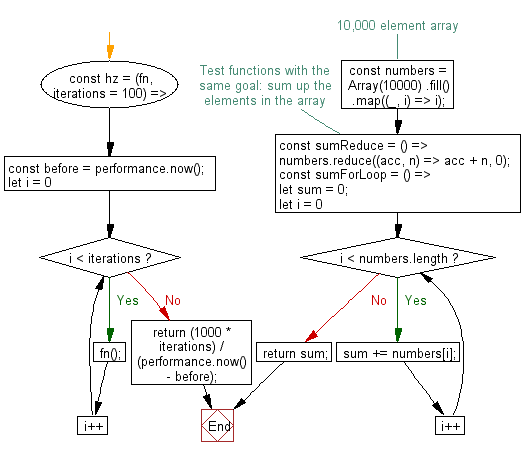# JavaScript: Get the number of times a function executed per second

## JavaScript fundamental (ES6 Syntax): Exercise-218 with Solution

Write a JavaScript program to get the number of times a function executed per second.

hz is the unit for hertz, the unit of frequency defined as one cycle per second.

• Use performance.now() to get the difference in milliseconds before and after the iteration loop to calculate the time elapsed executing the function iterations times.
• Return the number of cycles per second by converting milliseconds to seconds and dividing it by the time elapsed.
• Omit the second argument, iterations, to use the default of 100 iterations.

Sample Solution:

JavaScript Code:

``````//#Source https://bit.ly/2neWfJ2
const hz = (fn, iterations = 100) => {
const before = performance.now();
for (let i = 0; i < iterations; i++) fn();
return (1000 * iterations) / (performance.now() - before);
};
// 10,000 element array
const numbers = Array(10000)
.fill()
.map((_, i) => i);

// Test functions with the same goal: sum up the elements in the array
const sumReduce = () => numbers.reduce((acc, n) => acc + n, 0);
const sumForLoop = () => {
let sum = 0;
for (let i = 0; i < numbers.length; i++) sum += numbers[i];
return sum;
};

// sumForLoop` is nearly 10 times faster
console.log(Math.round(hz(sumReduce)));
console.log(Math.round(hz(sumForLoop)));
```
```

Sample Output:

```3584
2688
```

Flowchart:Live Demo:

See the Pen javascript-fundamental-exercise-218 by w3resource (@w3resource) on CodePen.

Improve this sample solution and post your code through Disqus

What is the difficulty level of this exercise?

Test your Programming skills with w3resource's quiz.

﻿

## JavaScript: Tips of the Day

Checking if a key exists in a JavaScript object?

Checking for undefined-ness is not an accurate way of testing whether a key exists. What if the key exists but the value is actually undefined?

```var obj = { key: undefined };
obj["key"] !== undefined // false, but the key exists!
```

You should instead use the in operator:

`"key" in obj // true, regardless of the actual value`

If you want to check if a key doesn't exist, remember to use parenthesis:

```!("key" in obj) // true if "key" doesn't exist in object
!"key" in obj   // ERROR!  Equivalent to "false in obj"
```

Or, if you want to particularly test for properties of the object instance (and not inherited properties), use hasOwnProperty:

```obj.hasOwnProperty("key") // true
```

Checking for undefined-ness is not an accurate way of testing whether a key exists. What if the key exists but the value is actually undefined? var obj = { key: undefined }; obj["key"] !== undefined // false, but the key exists! You should instead use the in operator: "key" in obj // true, regardless of the actual value If you want to check if a key doesn't exist, remember to use parenthesis: !("key" in obj) // true if "key" doesn't exist in object !"key" in obj // ERROR! Equivalent to "false in obj" Or, if you want to particularly test for properties of the object instance (and not inherited properties), use hasOwnProperty: obj.hasOwnProperty("key") // true For performance comparison between the methods that are in, hasOwnProperty and key is undefined.

Ref: https://bit.ly/2CFNp1X